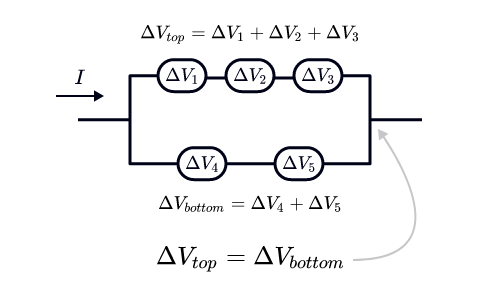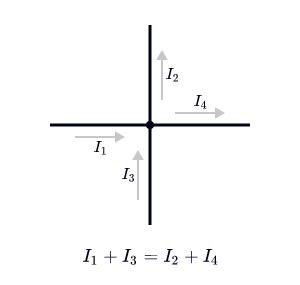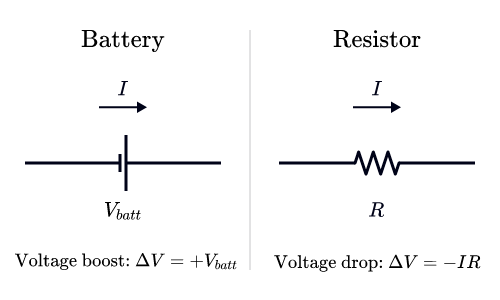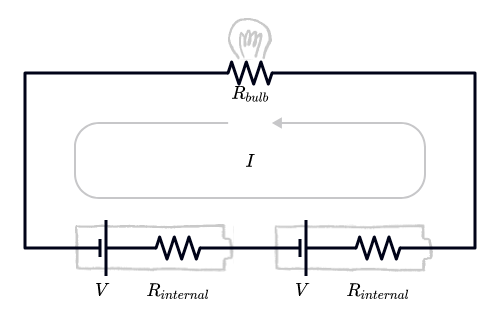Back

# Daily Challenge

## The Paths of Electricity

We are surrounded by all sorts of electric devices: some are simple like a light bulb, and some are very complicated like a power grid or a computer. Inside these devices, electricity flows around circuits, carrying energy precisely where it's needed.

Electric current is the coordinated motion of an unimaginable number of electrons, but the rules for how much current passes through a device are surprisingly straightforward.

Electric currents — flows of electric charge — are driven by differences in voltage. Current flows from positions of high voltage to positions of low voltage — similar to how water flows from high elevations to low elevations. In fact, voltage difference along a path in the circuit is the amount of potential energy per charge lost or gained.

Power sources like batteries are sources of voltage; they add potential energy to the moving charges. Resistors, like light bulbs for example, cause the voltage to drop quickly. To keep a steady current, the charges' potential energy splits up according to the circuit's resistance. Along wires, where resistance is low, the voltage barely varies at all. Each item in the circuit produces a change in the voltage, which we usually write $\Delta V$.

Whether you're looking at a simple circuit or a complicated nest of wires and bulbs with multiple batteries, there are two rules we can follow to piece together these $\Delta V$'s and figure out how much current is supplied.Voltage rule: The voltage must be consistent at any position, whichever way we calculate it. For example, if there is a circuit with two branches that come back together, the voltage across each branch must be the same.Current rule: In a steady state, charge cannot build up anywhere in the circuit. This means that if we look at any point in the circuit, the current flowing out must be the same as the current flowing in.

Armed with these rules, we just need some components to slot into our circuits. These components are the building blocks we can use to build any circuit, so engineers represent them with standard symbols. Two of the most important are batteries and resistors:• A battery (left) uses chemical energy to add potential energy to the charges flowing through it, maintaining a fixed $\Delta V$ between its ends (regardless of the current flowing through it).
• A resistor (right) obeys Ohm's law, which says the voltage drop $\Delta V$ across a resistor is proportional to the current $I$ flowing through it: $\Delta V = - I R$. The constant $R$ is called the resistance of the resistor.

How can we apply these rules? As an example, let's imagine we power a light bulb $($a resistor with resistance $R_\text{bulb})$ with two batteries that each provide a potential gain of $\Delta V=+V$. The batteries have internal resistance, which we represent with an extra resistor with resistance $R_\text{internal}$ connected in line with each ideal battery.According to the current law, the current is the same all the way around this loop. We can add up the $\Delta V$'s, starting at some arbitrary point P. When we get back to P, we should have the same voltage, meaning the sum of $\Delta V$'s must be zero. This gives us $V-IR_\text{internal}+V-IR_\text{internal}-IR_\text{bulb}=0.$We can rearrange this to get the total current around the circuit: $I=\frac{2V}{2R_\text{internal}+R_\text{bulb}}.$

# Today's Challenge

Suppose you take the batteries and wire them up to the bulb side by side, instead of in a line.

The brightness of the bulb is related to the current flowing through it $(I_1).$ If you completely remove one of the batteries, what is the new current $I$ through the bulb?

You can assume the resistance of the bulb is $5$ times larger than the internal resistance of each battery, i.e. $R_\text{bulb}=5R_\text{internal}.$

×